# Carnegie Mellon Bryant and OHallaron Computer Systems A

• Slides: 70Carnegie Mellon Bryant and O’Hallaron, Computer Systems: A Programmer’s Perspective, Third Edition 1Carnegie Mellon Code Optimization 15 -213/18 -213/14 -513/15 -513: Introduction to Computer Systems 10 th Lecture, September 27, 2018 Bryant and O’Hallaron, Computer Systems: A Programmer’s Perspective, Third Edition 2Carnegie Mellon Today ¢ ¢ Overview Generally Useful Optimizations § § ¢ Code motion/precomputation Strength reduction Sharing of common subexpressions Example: Bubblesort Optimization Blockers § Procedure calls § Memory aliasing ¢ ¢ Exploiting Instruction-Level Parallelism Dealing with Conditionals Bryant and O’Hallaron, Computer Systems: A Programmer’s Perspective, Third Edition 3Carnegie Mellon Performance Realities ¢ ¢ There’s more to performance than asymptotic complexity Constant factors matter too! § Easily see 10: 1 performance range depending on how code is written § Must optimize at multiple levels: § ¢ algorithm, data representations, procedures, and loops Must understand system to optimize performance § § How programs are compiled and executed How modern processors + memory systems operate How to measure program performance and identify bottlenecks How to improve performance without destroying code modularity and generality Bryant and O’Hallaron, Computer Systems: A Programmer’s Perspective, Third Edition 4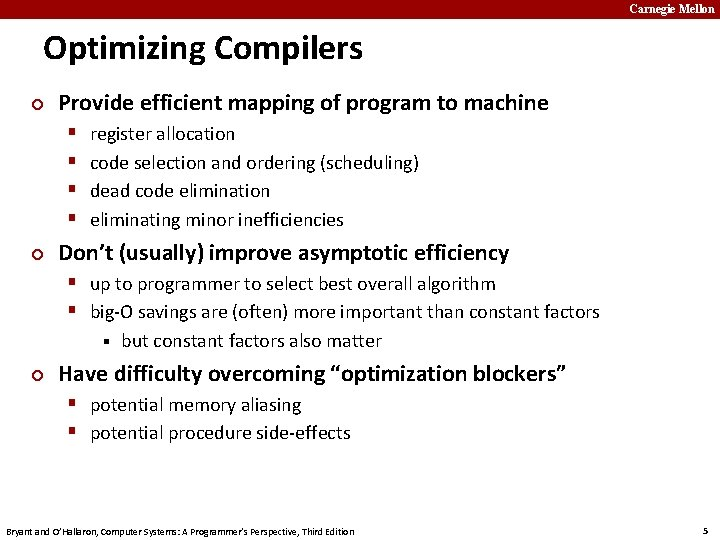Carnegie Mellon Optimizing Compilers ¢ Provide efficient mapping of program to machine § § ¢ register allocation code selection and ordering (scheduling) dead code elimination eliminating minor inefficiencies Don’t (usually) improve asymptotic efficiency § up to programmer to select best overall algorithm § big-O savings are (often) more important than constant factors § ¢ but constant factors also matter Have difficulty overcoming “optimization blockers” § potential memory aliasing § potential procedure side-effects Bryant and O’Hallaron, Computer Systems: A Programmer’s Perspective, Third Edition 5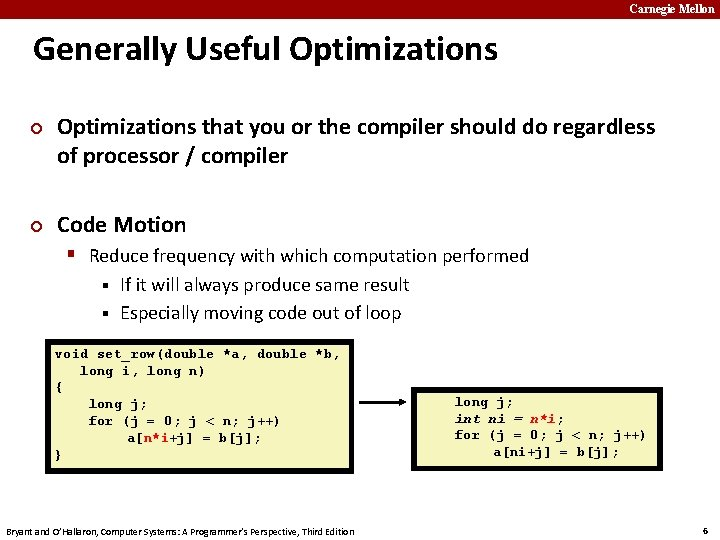Carnegie Mellon Generally Useful Optimizations ¢ ¢ Optimizations that you or the compiler should do regardless of processor / compiler Code Motion § Reduce frequency with which computation performed If it will always produce same result § Especially moving code out of loop § void set_row(double *a, double *b, long i, long n) { long j; for (j = 0; j < n; j++) a[n*i+j] = b[j]; } Bryant and O’Hallaron, Computer Systems: A Programmer’s Perspective, Third Edition long j; int ni = n*i; for (j = 0; j < n; j++) a[ni+j] = b[j]; 6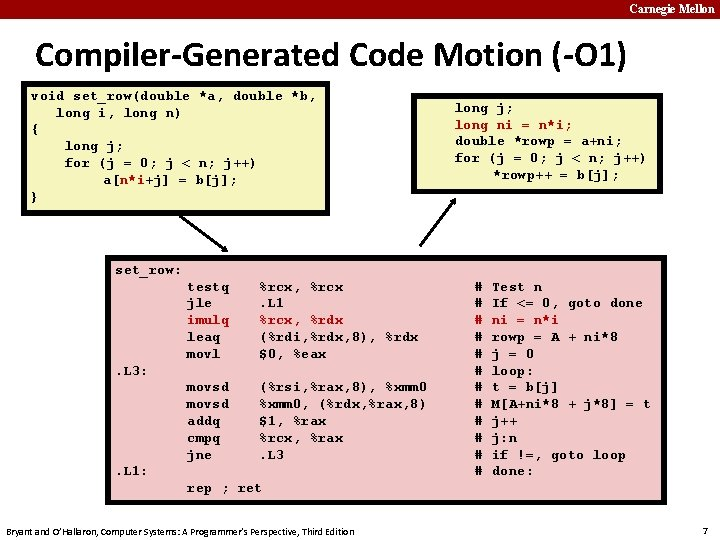Carnegie Mellon Compiler-Generated Code Motion (-O 1) void set_row(double *a, double *b, long i, long n) { long j; for (j = 0; j < n; j++) a[n*i+j] = b[j]; } long j; long ni = n*i; double *rowp = a+ni; for (j = 0; j < n; j++) *rowp++ = b[j]; set_row: testq jle imulq leaq movl %rcx, %rcx. L 1 %rcx, %rdx (%rdi, %rdx, 8), %rdx \$0, %eax movsd addq cmpq jne (%rsi, %rax, 8), %xmm 0, (%rdx, %rax, 8) \$1, %rax %rcx, %rax. L 3: . L 1: # # # Test n If <= 0, goto done ni = n*i rowp = A + ni*8 j = 0 loop: t = b[j] M[A+ni*8 + j*8] = t j++ j: n if !=, goto loop done: rep ; ret Bryant and O’Hallaron, Computer Systems: A Programmer’s Perspective, Third Edition 7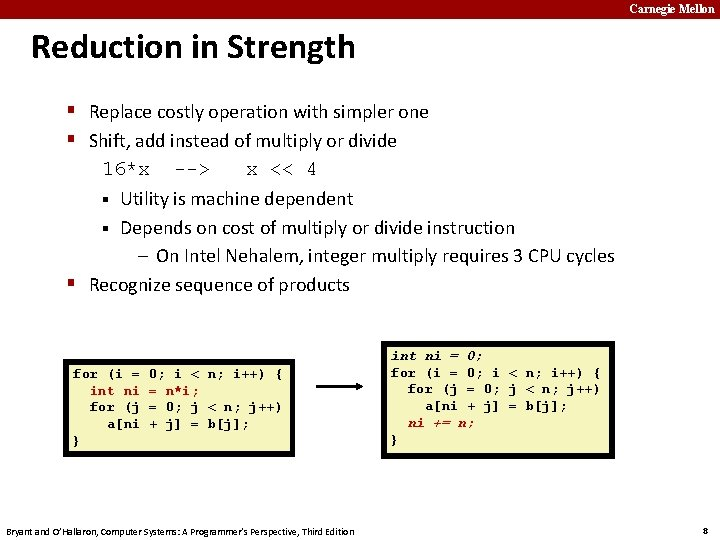Carnegie Mellon Reduction in Strength § Replace costly operation with simpler one § Shift, add instead of multiply or divide 16*x --> x << 4 § Utility is machine dependent § Depends on cost of multiply or divide instruction – On Intel Nehalem, integer multiply requires 3 CPU cycles § Recognize sequence of products for (i = int ni for (j a[ni } 0; i < n; i++) { = n*i; = 0; j < n; j++) + j] = b[j]; Bryant and O’Hallaron, Computer Systems: A Programmer’s Perspective, Third Edition int ni = 0; for (i = 0; i < n; i++) { for (j = 0; j < n; j++) a[ni + j] = b[j]; ni += n; } 8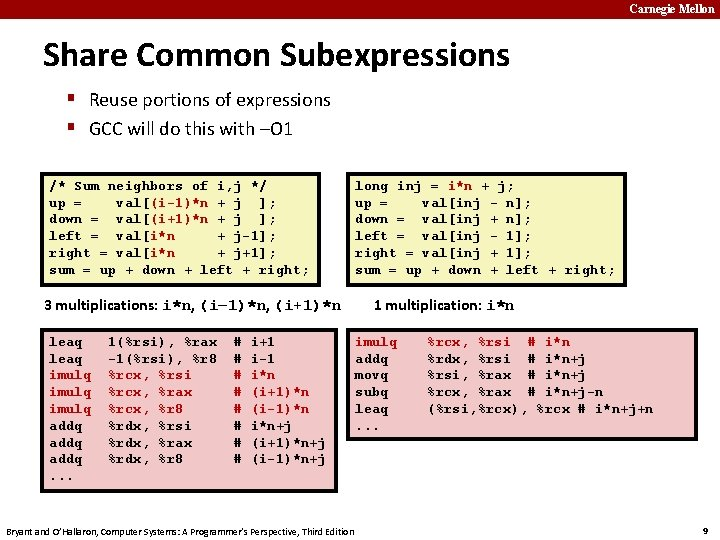Carnegie Mellon Share Common Subexpressions § Reuse portions of expressions § GCC will do this with –O 1 /* Sum neighbors of i, j */ up = val[(i-1)*n + j ]; down = val[(i+1)*n + j ]; left = val[i*n + j-1]; right = val[i*n + j+1]; sum = up + down + left + right; 3 multiplications: i*n, (i– 1)*n, (i+1)*n leaq imulq addq. . . 1(%rsi), %rax -1(%rsi), %r 8 %rcx, %rsi %rcx, %rax %rcx, %r 8 %rdx, %rsi %rdx, %rax %rdx, %r 8 # # # # i+1 i-1 i*n (i+1)*n (i-1)*n i*n+j (i+1)*n+j (i-1)*n+j Bryant and O’Hallaron, Computer Systems: A Programmer’s Perspective, Third Edition long inj = i*n + j; up = val[inj - n]; down = val[inj + n]; left = val[inj - 1]; right = val[inj + 1]; sum = up + down + left + right; 1 multiplication: i*n imulq addq movq subq leaq. . . %rcx, %rsi # i*n %rdx, %rsi # i*n+j %rsi, %rax # i*n+j %rcx, %rax # i*n+j-n (%rsi, %rcx), %rcx # i*n+j+n 9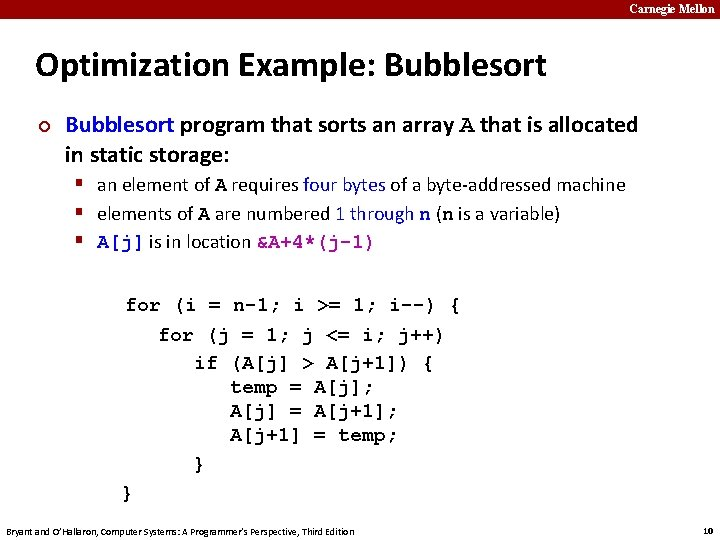Carnegie Mellon Optimization Example: Bubblesort ¢ Bubblesort program that sorts an array A that is allocated in static storage: § an element of A requires four bytes of a byte-addressed machine § elements of A are numbered 1 through n (n is a variable) § A[j] is in location &A+4*(j-1) for (i = n-1; i >= 1; i--) { for (j = 1; j <= i; j++) if (A[j] > A[j+1]) { temp = A[j]; A[j] = A[j+1]; A[j+1] = temp; } } Bryant and O’Hallaron, Computer Systems: A Programmer’s Perspective, Third Edition 10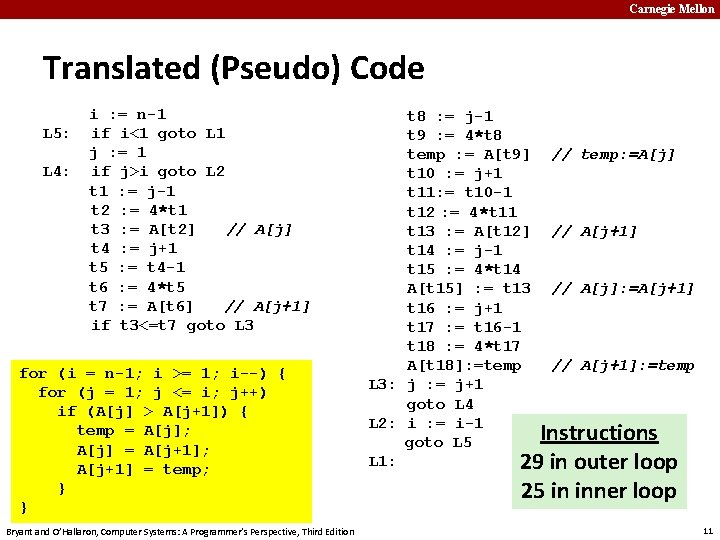Carnegie Mellon Translated (Pseudo) Code L 5: L 4: i : = n-1 if i<1 goto L 1 j : = 1 if j>i goto L 2 t 1 : = j-1 t 2 : = 4*t 1 t 3 : = A[t 2] // A[j] t 4 : = j+1 t 5 : = t 4 -1 t 6 : = 4*t 5 t 7 : = A[t 6] // A[j+1] if t 3<=t 7 goto L 3 for (i = n-1; i >= 1; i--) { for (j = 1; j <= i; j++) if (A[j] > A[j+1]) { temp = A[j]; A[j] = A[j+1]; A[j+1] = temp; } } Bryant and O’Hallaron, Computer Systems: A Programmer’s Perspective, Third Edition t 8 : = j-1 t 9 : = 4*t 8 temp : = A[t 9] // temp: =A[j] t 10 : = j+1 t 11: = t 10 -1 t 12 : = 4*t 11 t 13 : = A[t 12] // A[j+1] t 14 : = j-1 t 15 : = 4*t 14 A[t 15] : = t 13 // A[j]: =A[j+1] t 16 : = j+1 t 17 : = t 16 -1 t 18 : = 4*t 17 A[t 18]: =temp // A[j+1]: =temp L 3: j : = j+1 goto L 4 L 2: i : = i-1 Instructions goto L 5 L 1: 29 in outer loop 25 in inner loop 11Carnegie Mellon Redundancy in Address Calculation L 5: L 4: i : = n-1 if i<1 goto L 1 j : = 1 if j>i goto L 2 t 1 : = j-1 t 2 : = 4*t 1 t 3 : = A[t 2] // A[j] t 4 : = j+1 t 5 : = t 4 -1 t 6 : = 4*t 5 t 7 : = A[t 6] // A[j+1] if t 3<=t 7 goto L 3 Bryant and O’Hallaron, Computer Systems: A Programmer’s Perspective, Third Edition t 8 : =j-1 t 9 : = 4*t 8 temp : = A[t 9] t 10 : = j+1 t 11: = t 10 -1 t 12 : = 4*t 11 t 13 : = A[t 12] t 14 : = j-1 t 15 : = 4*t 14 A[t 15] : = t 13 t 16 : = j+1 t 17 : = t 16 -1 t 18 : = 4*t 17 A[t 18]: =temp L 3: j : = j+1 goto L 4 L 2: i : = i-1 goto L 5 L 1: // temp: =A[j] // A[j+1] // A[j]: =A[j+1] // A[j+1]: =temp 12Carnegie Mellon Redundancy Removed i : = n-1 L 5: if i<1 goto L 1 j : = 1 L 4: if j>i goto L 2 t 1 : = j-1 t 2 : = 4*t 1 t 3 : = A[t 2] // A[j] t 6 : = 4*j t 7 : = A[t 6] // A[j+1] if t 3<=t 7 goto L 3 t 8 : =j-1 t 9 : = 4*t 8 temp : = A[t 9] t 12 : = 4*j t 13 : = A[t 12] A[t 9]: = t 13 A[t 12]: =temp L 3: j : = j+1 goto L 4 L 2: i : = i-1 goto L 5 L 1: // temp: =A[j] // A[j+1] // A[j]: =A[j+1] // A[j+1]: =temp Instructions 20 in outer loop 16 in inner loop Bryant and O’Hallaron, Computer Systems: A Programmer’s Perspective, Third Edition 13Carnegie Mellon More Redundancy i : = n-1 L 5: if i<1 goto L 1 j : = 1 L 4: if j>i goto L 2 t 1 : = j-1 t 2 : = 4*t 1 t 3 : = A[t 2] // A[j] t 6 : = 4*j t 7 : = A[t 6] // A[j+1] if t 3<=t 7 goto L 3 Bryant and O’Hallaron, Computer Systems: A Programmer’s Perspective, Third Edition t 8 : =j-1 t 9 : = 4*t 8 temp : = A[t 9] t 12 : = 4*j t 13 : = A[t 12] A[t 9]: = t 13 A[t 12]: =temp L 3: j : = j+1 goto L 4 L 2: i : = i-1 goto L 5 L 1: // temp: =A[j] // A[j+1] // A[j]: =A[j+1] // A[j+1]: =temp 14Carnegie Mellon Redundancy Removed i : = n-1 A[t 2] : = t 7 L 5: if i<1 goto L 1 A[t 6] : = t 3 j : = 1 L 4: if j>i goto L 2 L 3: j : = j+1 t 1 : = j-1 goto L 4 t 2 : = 4*t 1 L 2: i : = i-1 t 3 : = A[t 2] // old_A[j] goto L 5 t 6 : = 4*j L 1: t 7 : = A[t 6] // A[j+1] if t 3<=t 7 goto L 3 // A[j]: =A[j+1] // A[j+1]: =old_A[j] Instructions 15 in outer loop 11 in inner loop Bryant and O’Hallaron, Computer Systems: A Programmer’s Perspective, Third Edition 15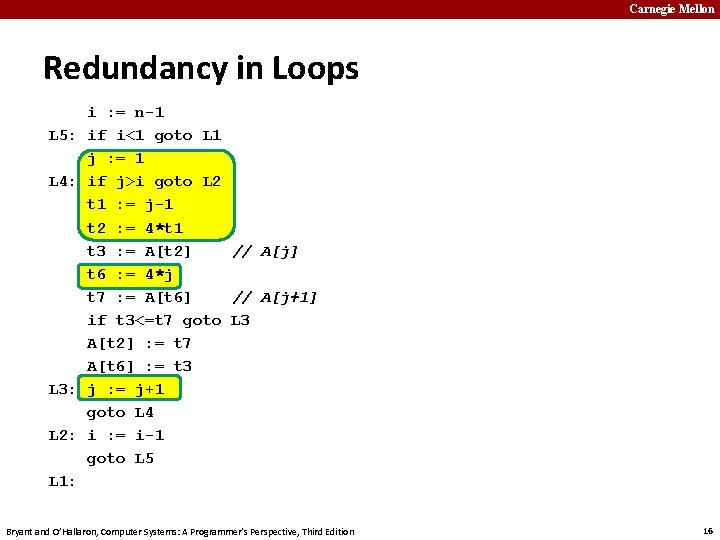Carnegie Mellon Redundancy in Loops L 5: L 4: L 3: L 2: i : = n-1 if i<1 goto L 1 j : = 1 if j>i goto L 2 t 1 : = j-1 t 2 : = 4*t 1 t 3 : = A[t 2] // A[j] t 6 : = 4*j t 7 : = A[t 6] // A[j+1] if t 3<=t 7 goto L 3 A[t 2] : = t 7 A[t 6] : = t 3 j : = j+1 goto L 4 i : = i-1 goto L 5 L 1: Bryant and O’Hallaron, Computer Systems: A Programmer’s Perspective, Third Edition 16Carnegie Mellon Redundancy Eliminated L 5: L 4: L 3: L 2: i : = n-1 if i<1 goto L 1 j : = 1 if j>i goto L 2 t 1 : = j-1 t 2 : = 4*t 1 t 3 : = A[t 2] // A[j] t 6 : = 4*j t 7 : = A[t 6] // A[j+1] if t 3<=t 7 goto L 3 A[t 2] : = t 7 A[t 6] : = t 3 j : = j+1 goto L 4 i : = i-1 goto L 5 L 1: Bryant and O’Hallaron, Computer Systems: A Programmer’s Perspective, Third Edition L 5: L 4: L 3: L 2: i : = n-1 if i<1 goto L 1 t 2 : = 0 t 6 : = 4 t 19 : = 4*i if t 6>t 19 goto L 2 t 3 : = A[t 2] t 7 : = A[t 6] if t 3<=t 7 goto L 3 A[t 2] : = t 7 A[t 6] : = t 3 t 2 : = t 2+4 t 6 : = t 6+4 goto L 4 i : = i-1 goto L 5 L 1: 17Carnegie Mellon Final Pseudo Code L 5: L 4: L 3: L 2: L 1: i : = n-1 Instruction Count if i<1 goto L 1 Before Optimizations t 2 : = 0 29 in outer loop t 6 : = 4 t 19 : = i << 2 25 in inner loop if t 6>t 19 goto L 2 t 3 : = A[t 2] t 7 : = A[t 6] Instruction Count if t 3<=t 7 goto L 3 After Optimizations A[t 2] : = t 7 15 in outer loop A[t 6] : = t 3 t 2 : = t 2+4 9 in inner loop t 6 : = t 6+4 goto L 4 i : = i-1 • These were Machine-Independent Optimizations. goto L 5 • Will be followed by Machine-Dependent Optimizations, including allocating temporaries to registers, converting to assembly code Bryant and O’Hallaron, Computer Systems: A Programmer’s Perspective, Third Edition 18Carnegie Mellon Today ¢ ¢ Overview Generally Useful Optimizations § § ¢ Code motion/precomputation Strength reduction Sharing of common subexpressions Example: Bubblesort Optimization Blockers § Procedure calls § Memory aliasing ¢ ¢ Exploiting Instruction-Level Parallelism Dealing with Conditionals Bryant and O’Hallaron, Computer Systems: A Programmer’s Perspective, Third Edition 19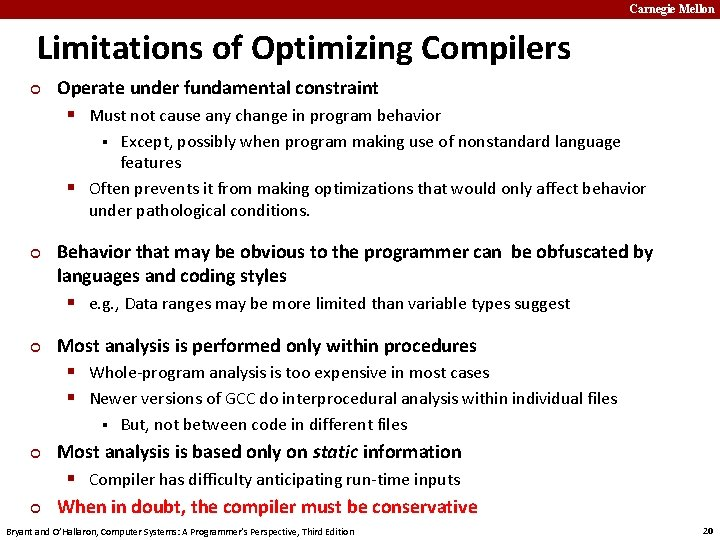Carnegie Mellon Limitations of Optimizing Compilers ¢ Operate under fundamental constraint § Must not cause any change in program behavior Except, possibly when program making use of nonstandard language features § Often prevents it from making optimizations that would only affect behavior under pathological conditions. § ¢ ¢ Behavior that may be obvious to the programmer can be obfuscated by languages and coding styles § e. g. , Data ranges may be more limited than variable types suggest Most analysis is performed only within procedures § Whole-program analysis is too expensive in most cases § Newer versions of GCC do interprocedural analysis within individual files § ¢ ¢ But, not between code in different files Most analysis is based only on static information § Compiler has difficulty anticipating run-time inputs When in doubt, the compiler must be conservative Bryant and O’Hallaron, Computer Systems: A Programmer’s Perspective, Third Edition 20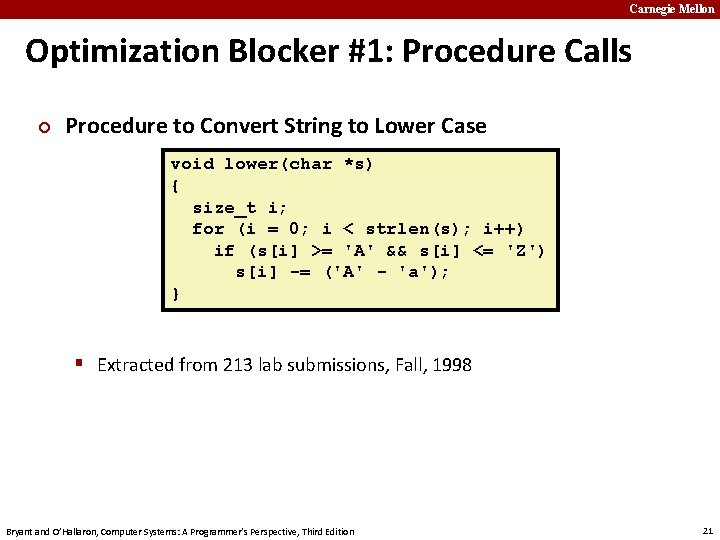Carnegie Mellon Optimization Blocker #1: Procedure Calls ¢ Procedure to Convert String to Lower Case void lower(char *s) { size_t i; for (i = 0; i < strlen(s); i++) if (s[i] >= 'A' && s[i] <= 'Z') s[i] -= ('A' - 'a'); } § Extracted from 213 lab submissions, Fall, 1998 Bryant and O’Hallaron, Computer Systems: A Programmer’s Perspective, Third Edition 21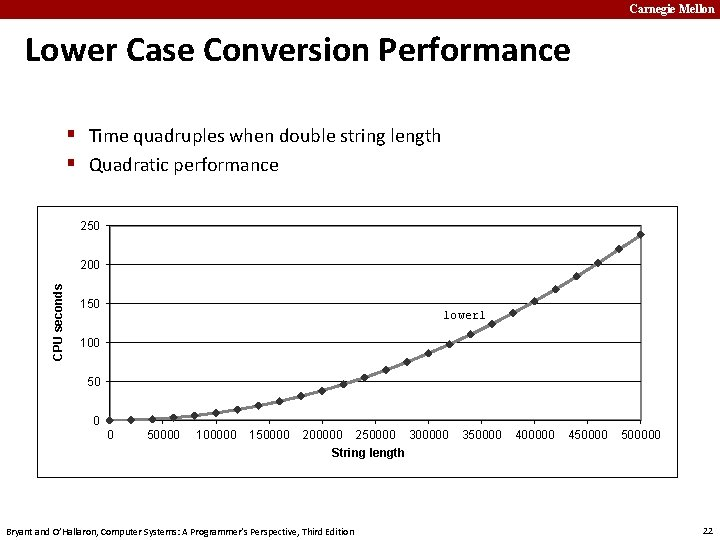Carnegie Mellon Lower Case Conversion Performance § Time quadruples when double string length § Quadratic performance 250 CPU seconds 200 150 lower 1 100 50 0 0 50000 100000 150000 200000 250000 300000 350000 400000 4500000 String length Bryant and O’Hallaron, Computer Systems: A Programmer’s Perspective, Third Edition 22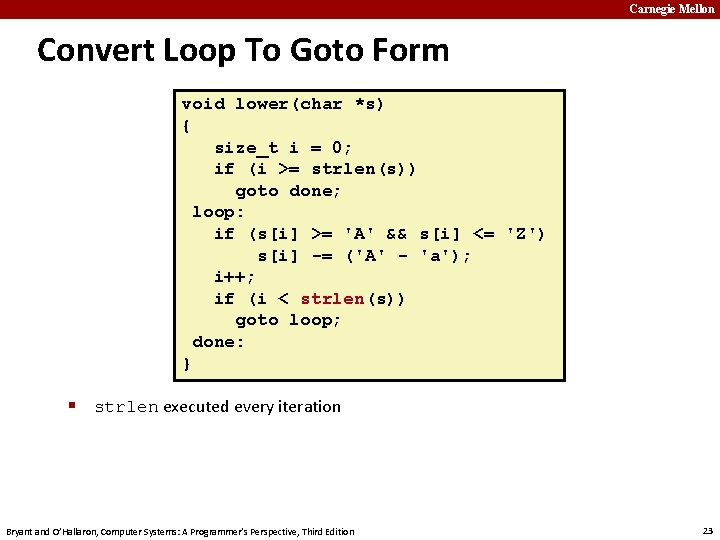Carnegie Mellon Convert Loop To Goto Form void lower(char *s) { size_t i = 0; if (i >= strlen(s)) goto done; loop: if (s[i] >= 'A' && s[i] <= 'Z') s[i] -= ('A' - 'a'); i++; if (i < strlen(s)) goto loop; done: } § strlen executed every iteration Bryant and O’Hallaron, Computer Systems: A Programmer’s Perspective, Third Edition 23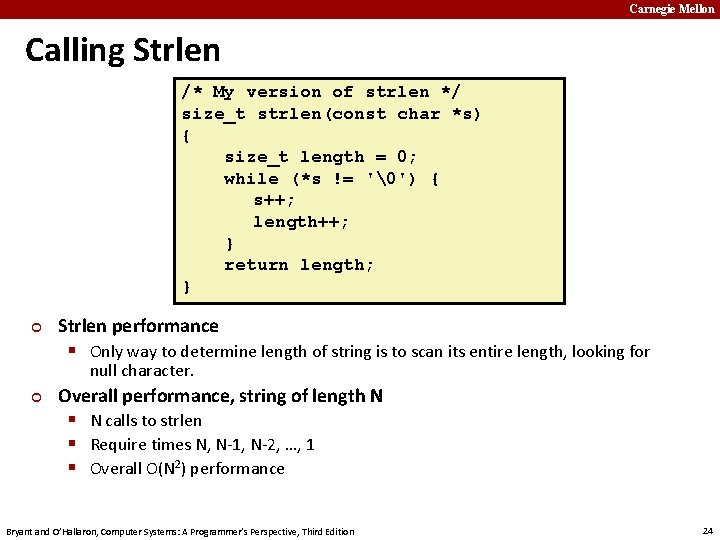Carnegie Mellon Calling Strlen /* My version of strlen */ size_t strlen(const char *s) { size_t length = 0; while (*s != '') { s++; length++; } return length; } ¢ Strlen performance § Only way to determine length of string is to scan its entire length, looking for null character. ¢ Overall performance, string of length N § N calls to strlen § Require times N, N-1, N-2, …, 1 § Overall O(N 2) performance Bryant and O’Hallaron, Computer Systems: A Programmer’s Perspective, Third Edition 24Carnegie Mellon Improving Performance void lower(char *s) { size_t i; size_t len = strlen(s); for (i = 0; i < len; i++) if (s[i] >= 'A' && s[i] <= 'Z') s[i] -= ('A' - 'a'); } § Move call to strlen outside of loop § Legal since result does not change from one iteration to another § Form of code motion Bryant and O’Hallaron, Computer Systems: A Programmer’s Perspective, Third Edition 25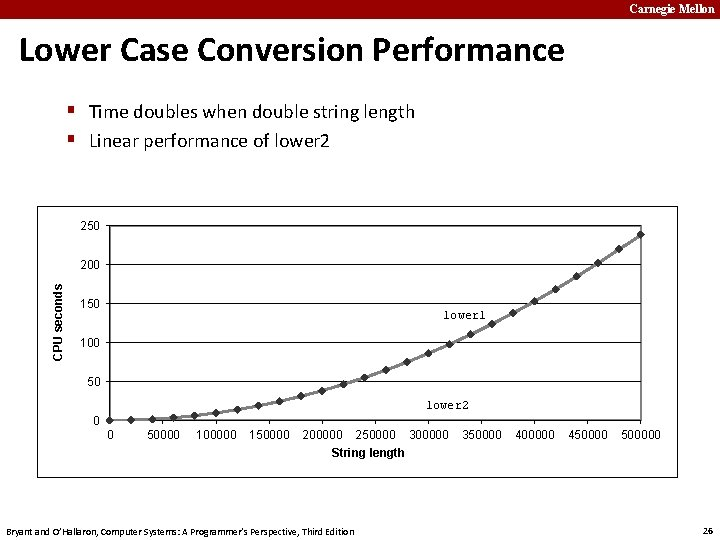Carnegie Mellon Lower Case Conversion Performance § Time doubles when double string length § Linear performance of lower 2 250 CPU seconds 200 150 lower 1 100 50 lower 2 0 0 50000 100000 150000 200000 250000 300000 350000 400000 4500000 String length Bryant and O’Hallaron, Computer Systems: A Programmer’s Perspective, Third Edition 26Carnegie Mellon Optimization Blocker: Procedure Calls ¢ Why couldn’t compiler move strlen out of inner loop? § Procedure may have side effects § Alters global state each time called § Function may not return same value for given arguments Depends on other parts of global state § Procedure lower could interact with strlen § ¢ ¢ Warning: § Compiler may treat procedure call as a black box § Weak optimizations near them size_t lencnt = 0; Remedies: § Use of inline functions GCC does this with –O 1 – Within single file § Do your own code motion § Bryant and O’Hallaron, Computer Systems: A Programmer’s Perspective, Third Edition size_t strlen(const char *s) { size_t length = 0; while (*s != '') { s++; length++; } lencnt += length; return length; } 27Carnegie Mellon Memory Matters /* Sum rows of n X n matrix a and store in vector b */ void sum_rows 1(double *a, double *b, long n) { long i, j; for (i = 0; i < n; i++) { b[i] = 0; for (j = 0; j < n; j++) b[i] += a[i*n + j]; } } # sum_rows 1 inner loop. L 4: movsd (%rsi, %rax, 8), %xmm 0 addsd (%rdi), %xmm 0 movsd %xmm 0, (%rsi, %rax, 8) addq \$8, %rdi cmpq %rcx, %rdi jne. L 4 # FP load # FP add # FP store § Code updates b[i] on every iteration § Why couldn’t compiler optimize this away? Bryant and O’Hallaron, Computer Systems: A Programmer’s Perspective, Third Edition 28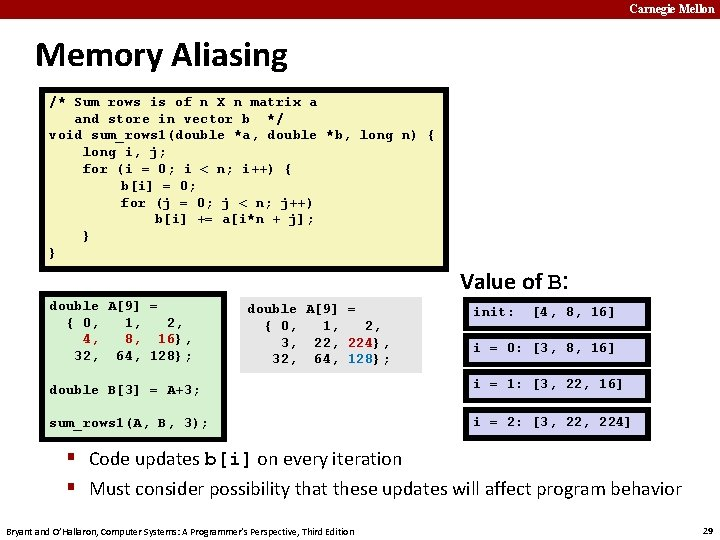Carnegie Mellon Memory Aliasing /* Sum rows is of n X n matrix a and store in vector b */ void sum_rows 1(double *a, double *b, long n) { long i, j; for (i = 0; i < n; i++) { b[i] = 0; for (j = 0; j < n; j++) b[i] += a[i*n + j]; } } Value of B: double A = { 0, 1, 2, 4, 8, 16}, 32, 64, 128}; double A = { 0, 1, 2, 3, 0, 22, 1, 3, 8, 224}, 0, 6, 16}, 96}, 32}, 0}, 32, 64, 128}; init: [4, 8, 16] i = 0: [3, 8, 16] double B = A+3; i = 1: [3, 22, 16] sum_rows 1(A, B, 3); i = 2: [3, 224] § Code updates b[i] on every iteration § Must consider possibility that these updates will affect program behavior Bryant and O’Hallaron, Computer Systems: A Programmer’s Perspective, Third Edition 29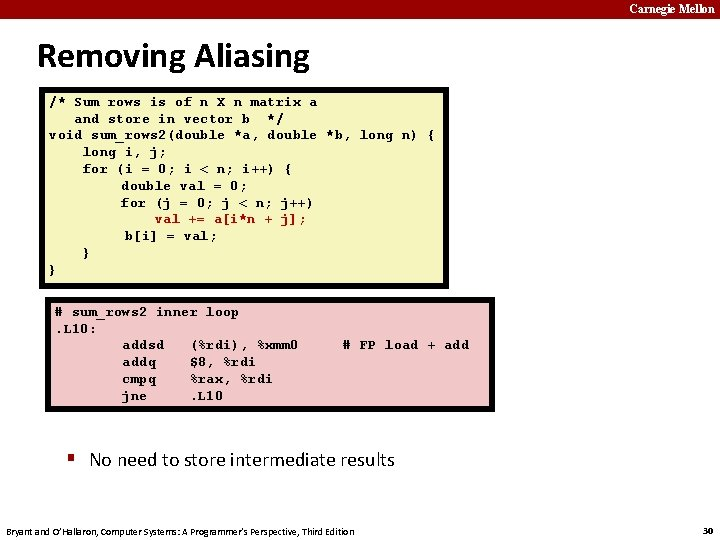Carnegie Mellon Removing Aliasing /* Sum rows is of n X n matrix a and store in vector b */ void sum_rows 2(double *a, double *b, long n) { long i, j; for (i = 0; i < n; i++) { double val = 0; for (j = 0; j < n; j++) val += a[i*n + j]; b[i] = val; } } # sum_rows 2 inner loop. L 10: addsd (%rdi), %xmm 0 addq \$8, %rdi cmpq %rax, %rdi jne. L 10 # FP load + add § No need to store intermediate results Bryant and O’Hallaron, Computer Systems: A Programmer’s Perspective, Third Edition 30Carnegie Mellon Optimization Blocker: Memory Aliasing ¢ Aliasing § Two different memory references specify single location § Easy to have happen in C Since allowed to do address arithmetic § Direct access to storage structures § Get in habit of introducing local variables § Accumulating within loops § Your way of telling compiler not to check for aliasing § Bryant and O’Hallaron, Computer Systems: A Programmer’s Perspective, Third Edition 31Carnegie Mellon Quiz Time! Check out: https: //canvas. cmu. edu/courses/5835 Bryant and O’Hallaron, Computer Systems: A Programmer’s Perspective, Third Edition 32Carnegie Mellon Today ¢ ¢ Overview Generally Useful Optimizations § § ¢ Code motion/precomputation Strength reduction Sharing of common subexpressions Example: Bubblesort Optimization Blockers § Procedure calls § Memory aliasing ¢ ¢ Exploiting Instruction-Level Parallelism Dealing with Conditionals Bryant and O’Hallaron, Computer Systems: A Programmer’s Perspective, Third Edition 33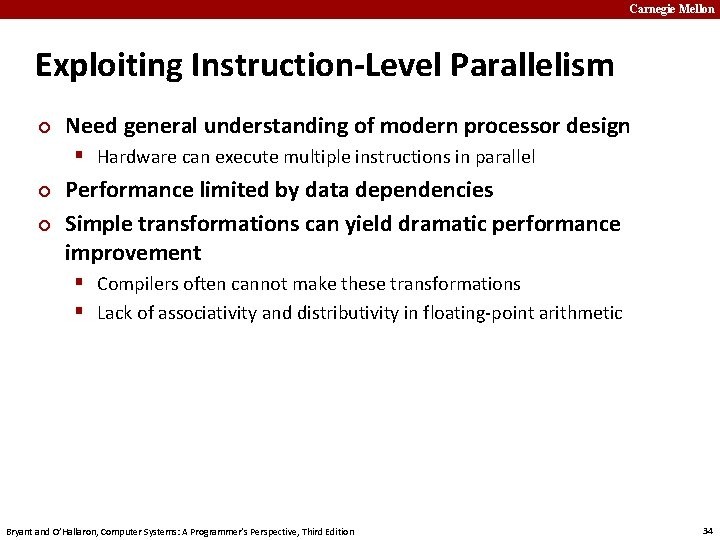Carnegie Mellon Exploiting Instruction-Level Parallelism ¢ Need general understanding of modern processor design § Hardware can execute multiple instructions in parallel ¢ ¢ Performance limited by data dependencies Simple transformations can yield dramatic performance improvement § Compilers often cannot make these transformations § Lack of associativity and distributivity in floating-point arithmetic Bryant and O’Hallaron, Computer Systems: A Programmer’s Perspective, Third Edition 34Carnegie Mellon Benchmark Example: Data Type for Vectors /* data structure for vectors */ typedef struct{ size_t len; data_t *data; } vec; ¢ Data Types § Use different declarations for data_t § § int long float double Bryant and O’Hallaron, Computer Systems: A Programmer’s Perspective, Third Edition len data 0 1 len-1 /* retrieve vector element and store at val */ int get_vec_element (*vec v, size_t idx, data_t *val) { if (idx >= v->len) return 0; *val = v->data[idx]; return 1; } 35Carnegie Mellon Benchmark Computation void combine 1(vec_ptr v, data_t *dest) { long int i; *dest = IDENT; for (i = 0; i < vec_length(v); i++) { data_t val; get_vec_element(v, i, &val); *dest = *dest OP val; } } ¢ Data Types ¢ Compute sum or product of vector elements Operations § Use different declarations § Use different definitions of § § § + / 0 § * / 1 for data_t int long float double Bryant and O’Hallaron, Computer Systems: A Programmer’s Perspective, Third Edition OP and IDENT 36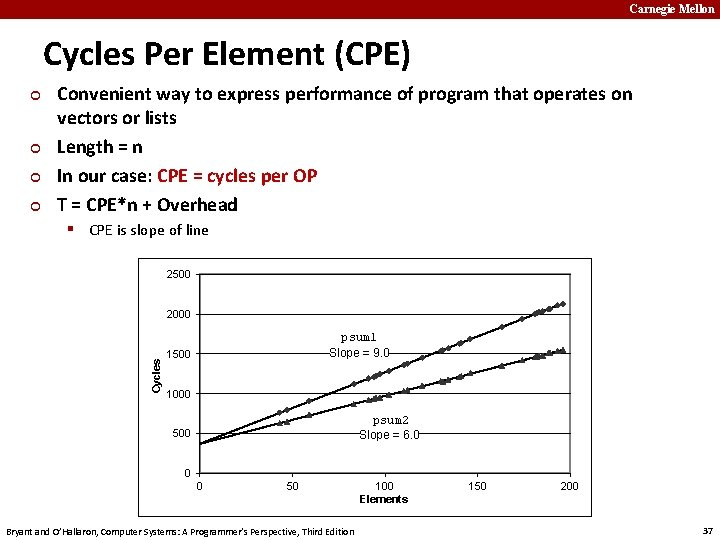Carnegie Mellon Cycles Per Element (CPE) ¢ ¢ Convenient way to express performance of program that operates on vectors or lists Length = n In our case: CPE = cycles per OP T = CPE*n + Overhead § CPE is slope of line 2500 Cycles 2000 psum 1 Slope = 9. 0 1500 1000 psum 2 Slope = 6. 0 500 0 0 50 Bryant and O’Hallaron, Computer Systems: A Programmer’s Perspective, Third Edition 100 Elements 150 200 37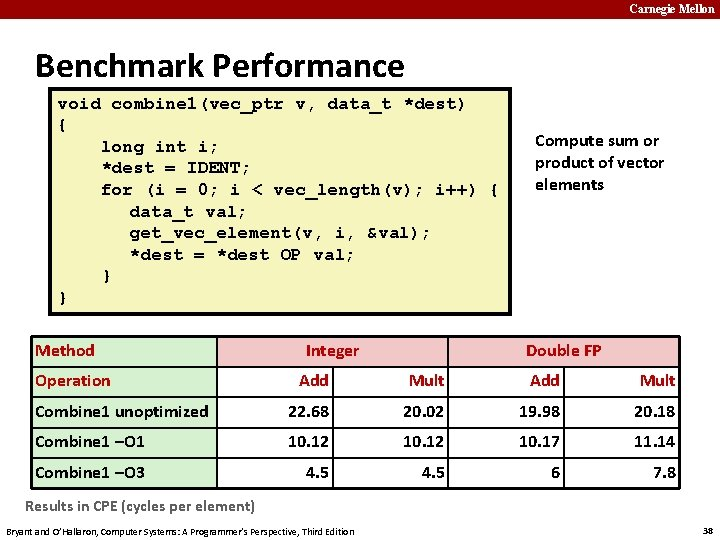Carnegie Mellon Benchmark Performance void combine 1(vec_ptr v, data_t *dest) { long int i; *dest = IDENT; for (i = 0; i < vec_length(v); i++) { data_t val; get_vec_element(v, i, &val); *dest = *dest OP val; } } Method Operation Integer Compute sum or product of vector elements Double FP Add Mult Combine 1 unoptimized 22. 68 20. 02 19. 98 20. 18 Combine 1 –O 1 10. 12 10. 17 11. 14 Combine 1 –O 3 4. 5 6 7. 8 Results in CPE (cycles per element) Bryant and O’Hallaron, Computer Systems: A Programmer’s Perspective, Third Edition 38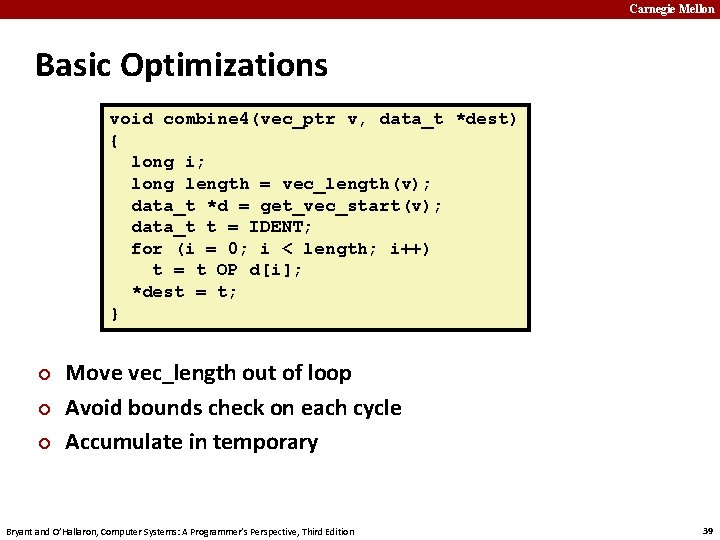Carnegie Mellon Basic Optimizations void combine 4(vec_ptr v, data_t *dest) { long i; long length = vec_length(v); data_t *d = get_vec_start(v); data_t t = IDENT; for (i = 0; i < length; i++) t = t OP d[i]; *dest = t; } ¢ ¢ ¢ Move vec_length out of loop Avoid bounds check on each cycle Accumulate in temporary Bryant and O’Hallaron, Computer Systems: A Programmer’s Perspective, Third Edition 39Carnegie Mellon Effect of Basic Optimizations void combine 4(vec_ptr v, data_t *dest) { long i; long length = vec_length(v); data_t *d = get_vec_start(v); data_t t = IDENT; for (i = 0; i < length; i++) t = t OP d[i]; *dest = t; } Method Operation Combine 1 –O 1 Combine 4 ¢ Integer Double FP Add Mult 10. 12 10. 17 11. 14 1. 27 3. 01 5. 01 Eliminates sources of overhead in loop Bryant and O’Hallaron, Computer Systems: A Programmer’s Perspective, Third Edition 40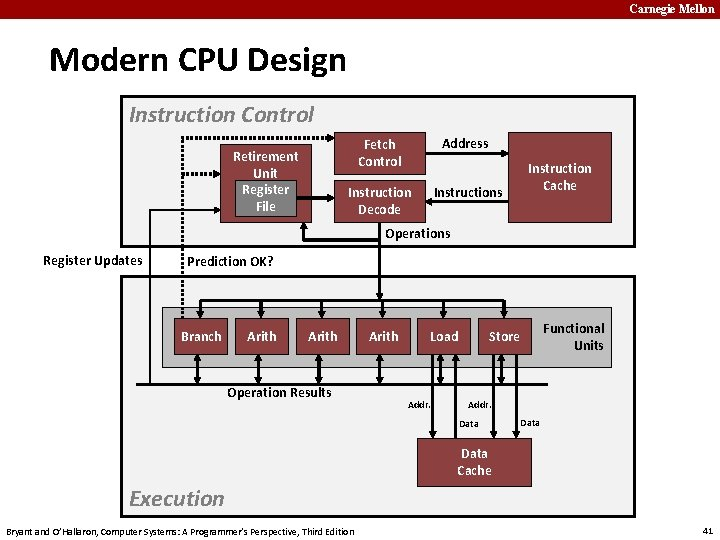Carnegie Mellon Modern CPU Design Instruction Control Retirement Unit Register File Fetch Control Address Instruction Decode Instructions Instruction Cache Operations Register Updates Prediction OK? Branch Arith Operation Results Arith Load Addr. Functional Units Store Addr. Data Cache Execution Bryant and O’Hallaron, Computer Systems: A Programmer’s Perspective, Third Edition 41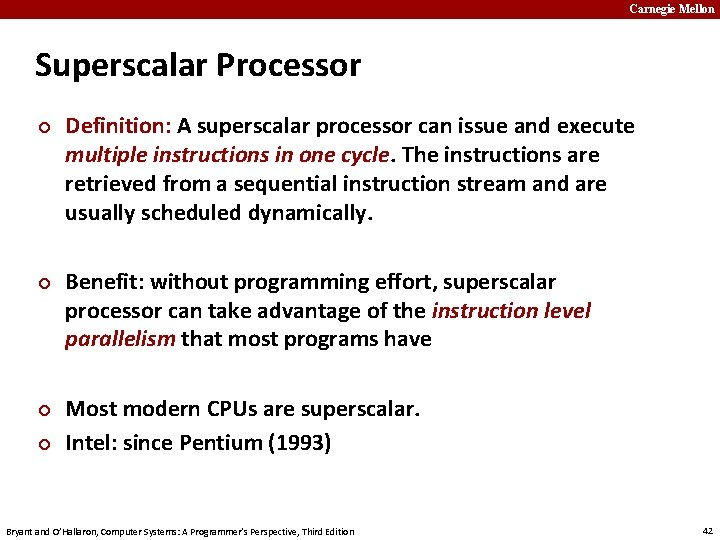Carnegie Mellon Superscalar Processor ¢ ¢ Definition: A superscalar processor can issue and execute multiple instructions in one cycle. The instructions are retrieved from a sequential instruction stream and are usually scheduled dynamically. Benefit: without programming effort, superscalar processor can take advantage of the instruction level parallelism that most programs have Most modern CPUs are superscalar. Intel: since Pentium (1993) Bryant and O’Hallaron, Computer Systems: A Programmer’s Perspective, Third Edition 42Carnegie Mellon Pipelined Functional Units Stage 1 long mult_eg(long a, long b, long c) { long p 1 = a*b; long p 2 = a*c; long p 3 = p 1 * p 2; return p 3; } Stage 2 Stage 3 Time Stage 1 Stage 2 Stage 3 § § 1 2 a*b a*c a*b 3 4 5 6 7 p 1*p 2 a*c a*b p 1*p 2 a*c p 1*p 2 Divide computation into stages Pass partial computations from stage to stage Stage i can start on new computation once values passed to i+1 E. g. , complete 3 multiplications in 7 cycles, even though each requires 3 cycles Bryant and O’Hallaron, Computer Systems: A Programmer’s Perspective, Third Edition 43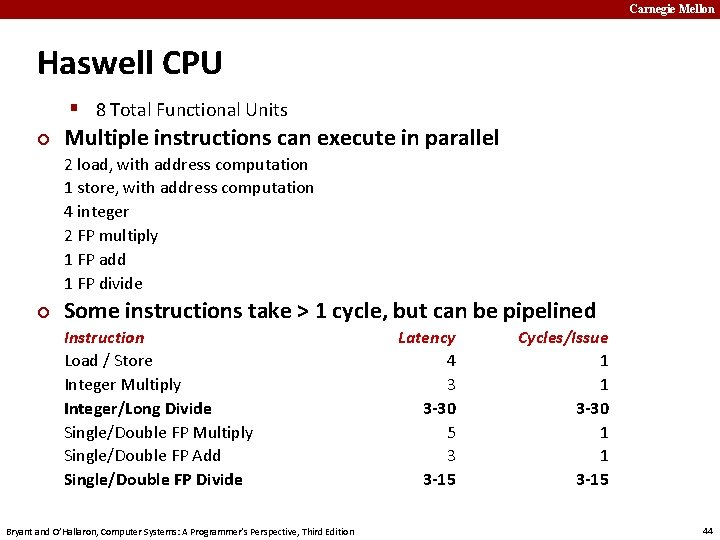Carnegie Mellon Haswell CPU § 8 Total Functional Units ¢ Multiple instructions can execute in parallel 2 load, with address computation 1 store, with address computation 4 integer 2 FP multiply 1 FP add 1 FP divide ¢ Some instructions take > 1 cycle, but can be pipelined Instruction Load / Store Integer Multiply Integer/Long Divide Single/Double FP Multiply Single/Double FP Add Single/Double FP Divide Bryant and O’Hallaron, Computer Systems: A Programmer’s Perspective, Third Edition Latency 4 3 3 -30 5 3 3 -15 Cycles/Issue 1 1 3 -30 1 1 3 -15 44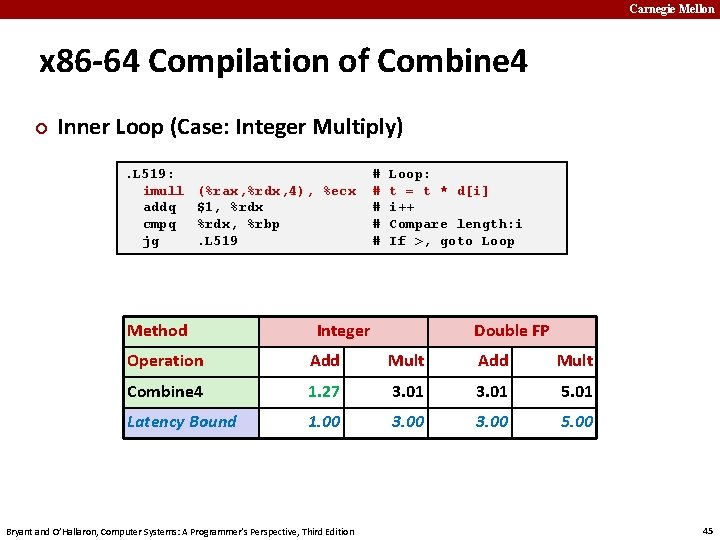Carnegie Mellon x 86 -64 Compilation of Combine 4 ¢ Inner Loop (Case: Integer Multiply). L 519: imull addq cmpq jg (%rax, %rdx, 4), %ecx \$1, %rdx, %rbp. L 519 Method # # # Loop: t = t * d[i] i++ Compare length: i If >, goto Loop Integer Double FP Operation Add Mult Combine 4 1. 27 3. 01 5. 01 Latency Bound 1. 00 3. 00 5. 00 Bryant and O’Hallaron, Computer Systems: A Programmer’s Perspective, Third Edition 45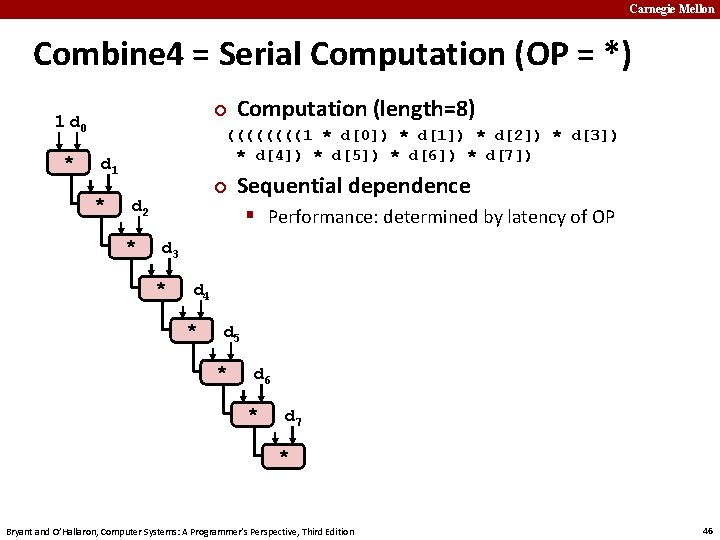Carnegie Mellon Combine 4 = Serial Computation (OP = *) ¢ 1 d 0 * ((((1 * d) * d) * d) * d) * d) * d) * d) * d) d 1 * Computation (length=8) ¢ d 2 * Sequential dependence § Performance: determined by latency of OP d 3 * d 4 * d 5 * d 6 * d 7 * Bryant and O’Hallaron, Computer Systems: A Programmer’s Perspective, Third Edition 46Carnegie Mellon Loop Unrolling (2 x 1) void unroll 2 a_combine(vec_ptr v, data_t *dest) { long length = vec_length(v); long limit = length-1; data_t *d = get_vec_start(v); data_t x = IDENT; long i; /* Combine 2 elements at a time */ for (i = 0; i < limit; i+=2) { x = (x OP d[i]) OP d[i+1]; } /* Finish any remaining elements */ for (; i < length; i++) { x = x OP d[i]; } *dest = x; } ¢ Perform 2 x more useful work per iteration Bryant and O’Hallaron, Computer Systems: A Programmer’s Perspective, Third Edition 47Carnegie Mellon Effect of Loop Unrolling Method ¢ Integer Operation Add Mult Combine 4 1. 27 3. 01 5. 01 Unroll 2 x 1 1. 01 3. 01 5. 01 Latency Bound 1. 00 3. 00 5. 00 Helps integer add § Achieves latency bound ¢ Double FP x = (x OP d[i]) OP d[i+1]; Others don’t improve. Why? § Still sequential dependency Bryant and O’Hallaron, Computer Systems: A Programmer’s Perspective, Third Edition 48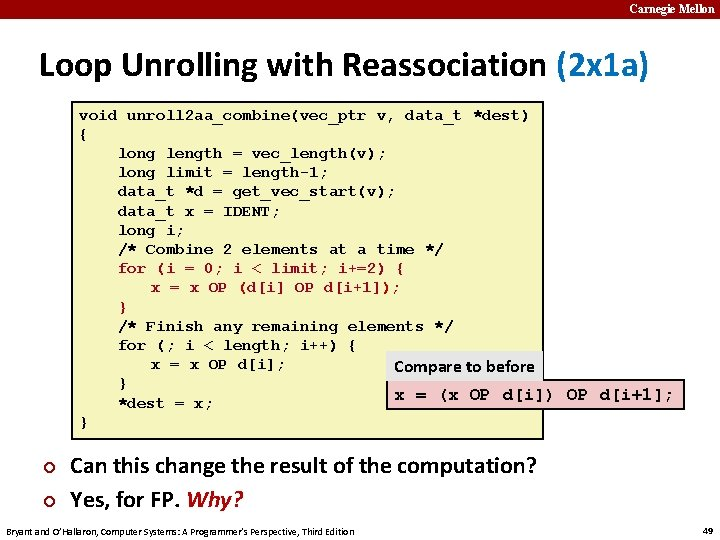Carnegie Mellon Loop Unrolling with Reassociation (2 x 1 a) void unroll 2 aa_combine(vec_ptr v, data_t *dest) { long length = vec_length(v); long limit = length-1; data_t *d = get_vec_start(v); data_t x = IDENT; long i; /* Combine 2 elements at a time */ for (i = 0; i < limit; i+=2) { x = x OP (d[i] OP d[i+1]); } /* Finish any remaining elements */ for (; i < length; i++) { x = x OP d[i]; Compare to before } x = (x OP d[i]) OP d[i+1]; *dest = x; } ¢ ¢ Can this change the result of the computation? Yes, for FP. Why? Bryant and O’Hallaron, Computer Systems: A Programmer’s Perspective, Third Edition 49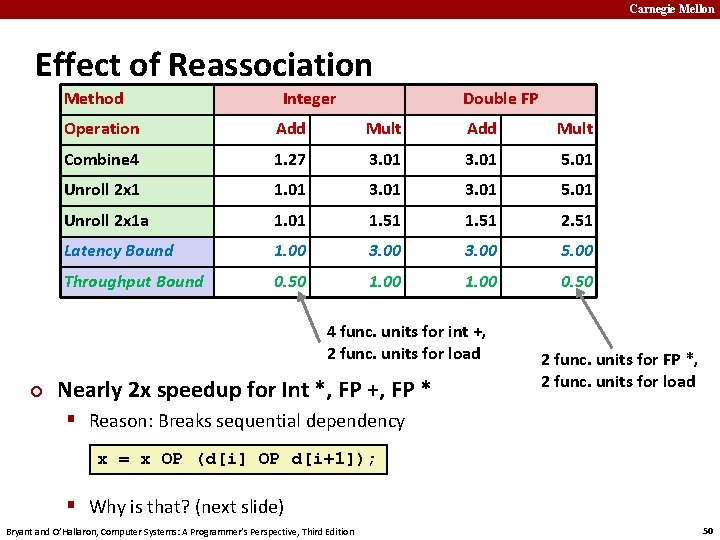Carnegie Mellon Effect of Reassociation Method Integer Double FP Operation Add Mult Combine 4 1. 27 3. 01 5. 01 Unroll 2 x 1 1. 01 3. 01 5. 01 Unroll 2 x 1 a 1. 01 1. 51 2. 51 Latency Bound 1. 00 3. 00 5. 00 Throughput Bound 0. 50 1. 00 0. 50 4 func. units for int +, 2 func. units for load ¢ Nearly 2 x speedup for Int *, FP +, FP * 2 func. units for FP *, 2 func. units for load § Reason: Breaks sequential dependency x = x OP (d[i] OP d[i+1]); § Why is that? (next slide) Bryant and O’Hallaron, Computer Systems: A Programmer’s Perspective, Third Edition 50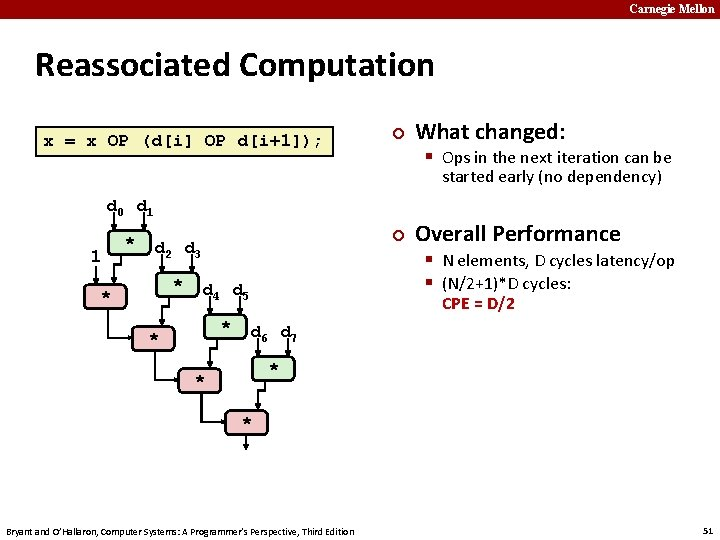Carnegie Mellon Reassociated Computation x = x OP (d[i] OP d[i+1]); ¢ What changed: § Ops in the next iteration can be started early (no dependency) d 0 d 1 1 * ¢ d 2 d 3 * * § N elements, D cycles latency/op § (N/2+1)*D cycles: d 4 d 5 * * Overall Performance CPE = D/2 d 6 d 7 * * * Bryant and O’Hallaron, Computer Systems: A Programmer’s Perspective, Third Edition 51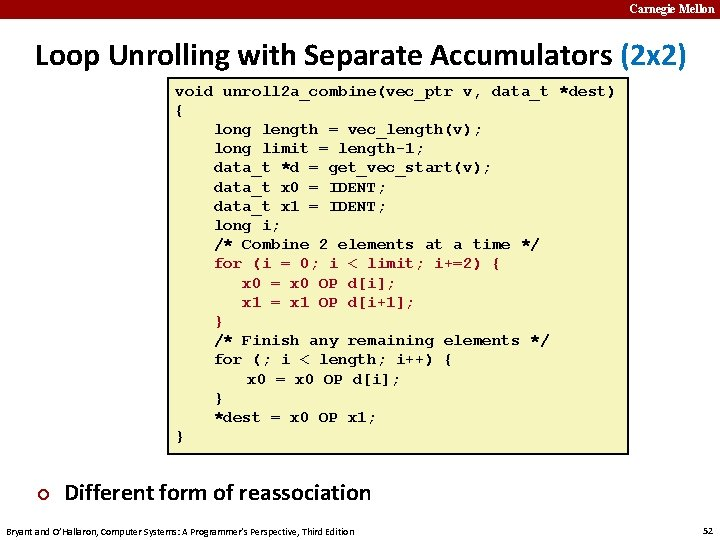Carnegie Mellon Loop Unrolling with Separate Accumulators (2 x 2) void unroll 2 a_combine(vec_ptr v, data_t *dest) { long length = vec_length(v); long limit = length-1; data_t *d = get_vec_start(v); data_t x 0 = IDENT; data_t x 1 = IDENT; long i; /* Combine 2 elements at a time */ for (i = 0; i < limit; i+=2) { x 0 = x 0 OP d[i]; x 1 = x 1 OP d[i+1]; } /* Finish any remaining elements */ for (; i < length; i++) { x 0 = x 0 OP d[i]; } *dest = x 0 OP x 1; } ¢ Different form of reassociation Bryant and O’Hallaron, Computer Systems: A Programmer’s Perspective, Third Edition 52Carnegie Mellon Effect of Separate Accumulators Method Integer Double FP Operation Add Mult Combine 4 1. 27 3. 01 5. 01 Unroll 2 x 1 1. 01 3. 01 5. 01 Unroll 2 x 1 a 1. 01 1. 51 2. 51 Unroll 2 x 2 0. 81 1. 51 2. 51 Latency Bound 1. 00 3. 00 5. 00 Throughput Bound 0. 50 1. 00 0. 50 ¢ Int + makes use of two load units x 0 = x 0 OP d[i]; x 1 = x 1 OP d[i+1]; ¢ 2 x speedup (over unroll 2) for Int *, FP +, FP * Bryant and O’Hallaron, Computer Systems: A Programmer’s Perspective, Third Edition 53Carnegie Mellon Separate Accumulators x 0 = x 0 OP d[i]; x 1 = x 1 OP d[i+1]; 1 d 0 1 d 1 * * d 2 * operations ¢ * d 6 What changed: § Two independent “streams” of d 3 d 4 * ¢ § N elements, D cycles latency/op § Should be (N/2+1)*D cycles: d 5 * * Overall Performance d 7 * * Bryant and O’Hallaron, Computer Systems: A Programmer’s Perspective, Third Edition CPE = D/2 § CPE matches prediction! What Now? 54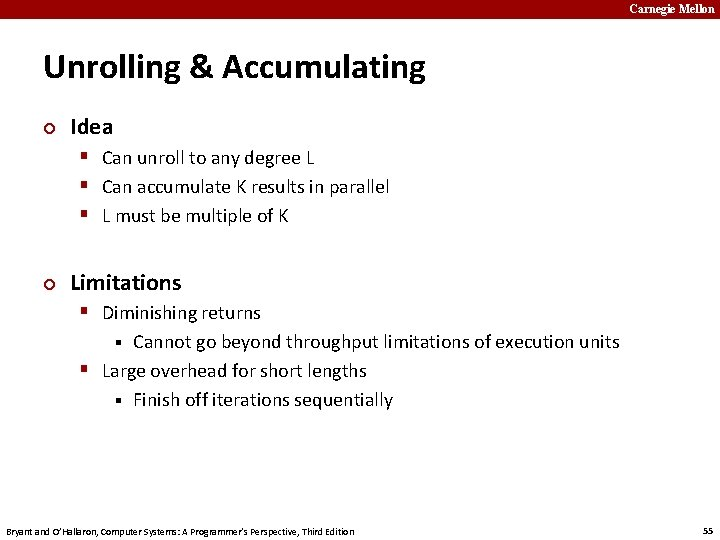Carnegie Mellon Unrolling & Accumulating ¢ Idea § Can unroll to any degree L § Can accumulate K results in parallel § L must be multiple of K ¢ Limitations § Diminishing returns Cannot go beyond throughput limitations of execution units § Large overhead for short lengths § Finish off iterations sequentially § Bryant and O’Hallaron, Computer Systems: A Programmer’s Perspective, Third Edition 55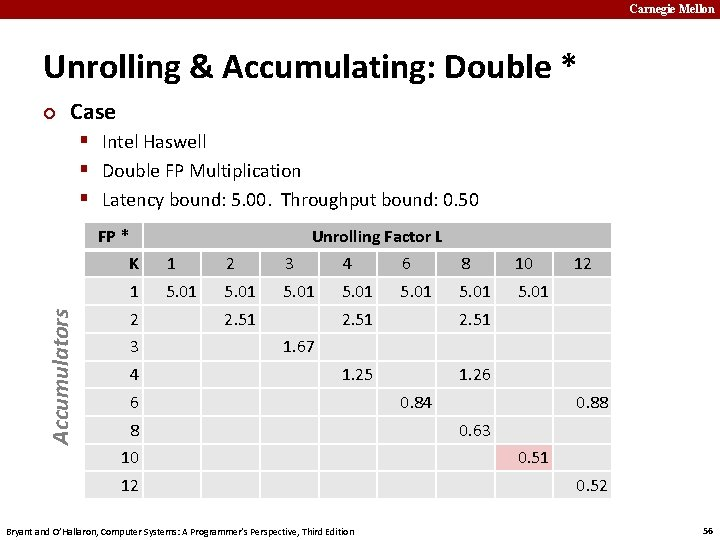Carnegie Mellon Unrolling & Accumulating: Double * ¢ Case § Intel Haswell § Double FP Multiplication § Latency bound: 5. 00. Throughput bound: 0. 50 Accumulators FP * Unrolling Factor L K 1 2 3 4 6 8 10 1 5. 01 5. 01 2 3 4 2. 51 1. 25 1. 26 12 1. 67 6 8 10 12 Bryant and O’Hallaron, Computer Systems: A Programmer’s Perspective, Third Edition 0. 84 0. 88 0. 63 0. 51 0. 52 56Carnegie Mellon Achievable Performance Method Integer Double FP Operation Add Mult Best 0. 54 1. 01 0. 52 Latency Bound 1. 00 3. 00 5. 00 Throughput Bound 0. 50 1. 00 0. 50 ¢ ¢ Limited only by throughput of functional units Up to 42 X improvement over original, unoptimized code Bryant and O’Hallaron, Computer Systems: A Programmer’s Perspective, Third Edition 58Programming with AVX 2 Carnegie Mellon YMM Registers n 16 total, each 32 bytes n 32 single-byte integers n 16 16 -bit integers n 8 32 -bit integers n 8 single-precision floats n 4 double-precision floats n 1 single-precision float n 1 double-precision float Bryant and O’Hallaron, Computer Systems: A Programmer’s Perspective, Third Edition 59Carnegie Mellon SIMD Operations: Single Precision vaddps %ymm 0, %ymm 1 %ymm 0 + + + + %ymm 1 n SIMD Operations: Double Precision vaddpd %ymm 0, %ymm 1 %ymm 0 + + %ymm 1 Bryant and O’Hallaron, Computer Systems: A Programmer’s Perspective, Third Edition 60Carnegie Mellon Using Vector Instructions Method Integer Double FP Operation Add Mult Scalar Best 0. 54 1. 01 0. 52 Vector Best 0. 06 0. 24 0. 25 0. 16 Latency Bound 0. 50 3. 00 5. 00 Throughput Bound 0. 50 1. 00 0. 50 Vec Throughput Bound 0. 06 0. 12 0. 25 0. 12 ¢ Make use of AVX Instructions § Parallel operations on multiple data elements § See Web Aside OPT: SIMD on CS: APP web page Bryant and O’Hallaron, Computer Systems: A Programmer’s Perspective, Third Edition 61Carnegie Mellon What About Branches? ¢ Challenge § Instruction Control Unit must work well ahead of Execution Unit to generate enough operations to keep EU busy 404663: 404668: 40466 b: 40466 d: mov cmp jge mov \$0 x 0, %eax (%rdi), %rsi 404685 0 x 8(%rdi), %rax Executing How to continue? . . . 404685: repz retq § When encounters conditional branch, cannot reliably determine where to continue fetching Bryant and O’Hallaron, Computer Systems: A Programmer’s Perspective, Third Edition 62Carnegie Mellon Modern CPU Design Instruction Control Retirement Unit Register File Fetch Control Address Instruction Decode Instructions Instruction Cache Operations Register Updates Prediction OK? Branch Arith Operation Results Arith Load Addr. Functional Units Store Addr. Data Cache Execution Bryant and O’Hallaron, Computer Systems: A Programmer’s Perspective, Third Edition 63Carnegie Mellon Branch Outcomes § When encounter conditional branch, cannot determine where to continue fetching § Branch Taken: Transfer control to branch target § Branch Not-Taken: Continue with next instruction in sequence § Cannot resolve until outcome determined by branch/integer unit 404663: 404668: 40466 b: 40466 d: mov cmp jge mov \$0 x 0, %eax (%rdi), %rsi 404685 0 x 8(%rdi), %rax Branch Taken . . . 404685: Branch Not-Taken repz retq Bryant and O’Hallaron, Computer Systems: A Programmer’s Perspective, Third Edition 64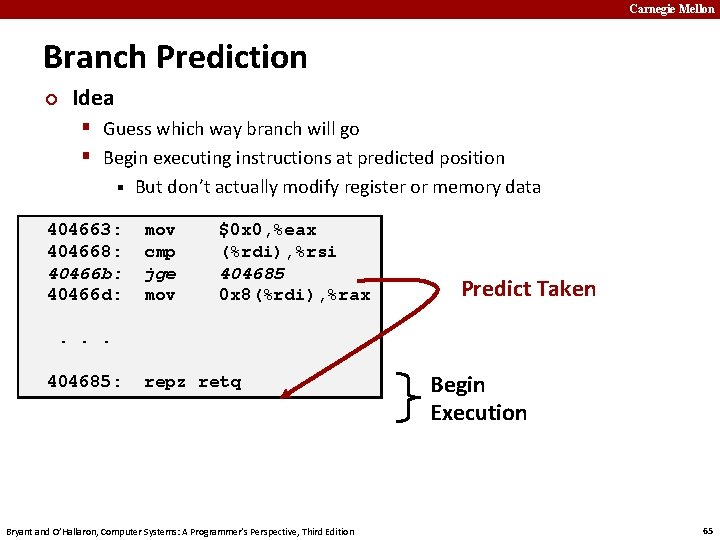Carnegie Mellon Branch Prediction ¢ Idea § Guess which way branch will go § Begin executing instructions at predicted position § 404663: 404668: 40466 b: 40466 d: But don’t actually modify register or memory data mov cmp jge mov \$0 x 0, %eax (%rdi), %rsi 404685 0 x 8(%rdi), %rax Predict Taken . . . 404685: repz retq Bryant and O’Hallaron, Computer Systems: A Programmer’s Perspective, Third Edition Begin Execution 65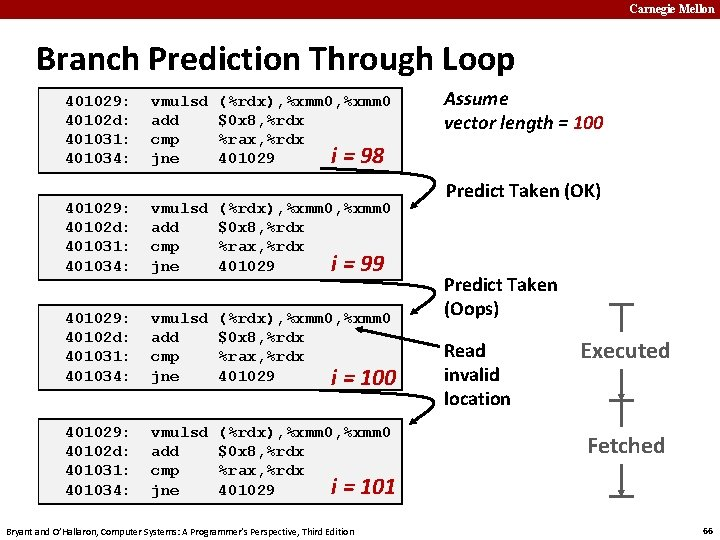Carnegie Mellon Branch Prediction Through Loop 401029: 40102 d: 401031: 401034: vmulsd add cmp jne (%rdx), %xmm 0 \$0 x 8, %rdx %rax, %rdx i = 98 401029: 40102 d: 401031: 401034: vmulsd add cmp jne (%rdx), %xmm 0 \$0 x 8, %rdx %rax, %rdx i = 99 401029: 40102 d: 401031: 401034: vmulsd add cmp jne (%rdx), %xmm 0 \$0 x 8, %rdx %rax, %rdx 401029 i = 100 401029: 40102 d: 401031: 401034: vmulsd add cmp jne (%rdx), %xmm 0 \$0 x 8, %rdx %rax, %rdx i = 101 401029 Bryant and O’Hallaron, Computer Systems: A Programmer’s Perspective, Third Edition Assume vector length = 100 Predict Taken (OK) Predict Taken (Oops) Read invalid location Executed Fetched 66Carnegie Mellon Branch Misprediction Invalidation 401029: 40102 d: 401031: 401034: vmulsd add cmp jne (%rdx), %xmm 0 \$0 x 8, %rdx %rax, %rdx i = 98 401029: 40102 d: 401031: 401034: vmulsd add cmp jne (%rdx), %xmm 0 \$0 x 8, %rdx %rax, %rdx i = 99 401029: 40102 d: 401031: 401034: vmulsd add cmp jne (%rdx), %xmm 0 \$0 x 8, %rdx %rax, %rdx 401029 i = 100 Assume vector length = 100 Predict Taken (OK) Predict Taken (Oops) Invalidate 401029: 40102 d: 401031: 401034: vmulsd add cmp jne (%rdx), %xmm 0 \$0 x 8, %rdx %rax, %rdx i = 101 401029 Bryant and O’Hallaron, Computer Systems: A Programmer’s Perspective, Third Edition 67Carnegie Mellon Branch Misprediction Recovery 401029: 40102 d: 401031: 401034: 401036: . . . 401040: ¢ vmulsd add cmp jne jmp (%rdx), %xmm 0 \$0 x 8, %rdx i= %rax, %rdx 401029 401040 99 vmovsd %xmm 0, (%r 12) Definitely not taken Reload Pipeline Performance Cost § Multiple clock cycles on modern processor § Can be a major performance limiter Bryant and O’Hallaron, Computer Systems: A Programmer’s Perspective, Third Edition 68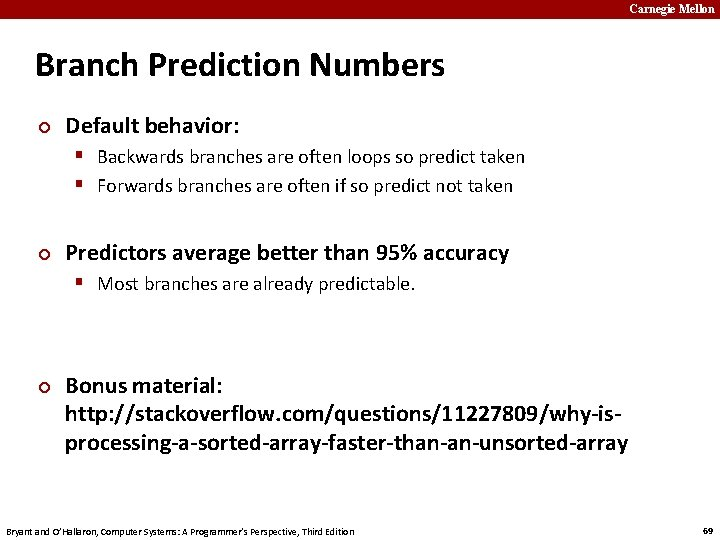Carnegie Mellon Branch Prediction Numbers ¢ Default behavior: § Backwards branches are often loops so predict taken § Forwards branches are often if so predict not taken ¢ Predictors average better than 95% accuracy § Most branches are already predictable. ¢ Bonus material: http: //stackoverflow. com/questions/11227809/why-isprocessing-a-sorted-array-faster-than-an-unsorted-array Bryant and O’Hallaron, Computer Systems: A Programmer’s Perspective, Third Edition 69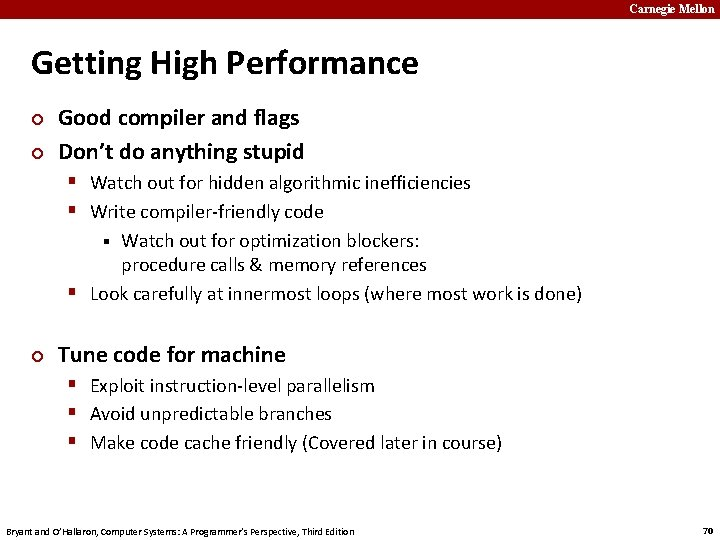Carnegie Mellon Getting High Performance ¢ ¢ Good compiler and flags Don’t do anything stupid § Watch out for hidden algorithmic inefficiencies § Write compiler-friendly code Watch out for optimization blockers: procedure calls & memory references § Look carefully at innermost loops (where most work is done) § ¢ Tune code for machine § Exploit instruction-level parallelism § Avoid unpredictable branches § Make code cache friendly (Covered later in course) Bryant and O’Hallaron, Computer Systems: A Programmer’s Perspective, Third Edition 70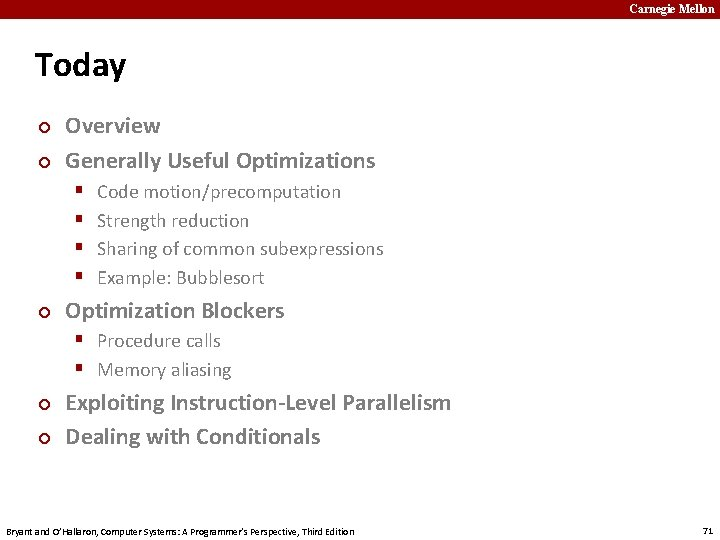Carnegie Mellon Today ¢ ¢ Overview Generally Useful Optimizations § § ¢ Code motion/precomputation Strength reduction Sharing of common subexpressions Example: Bubblesort Optimization Blockers § Procedure calls § Memory aliasing ¢ ¢ Exploiting Instruction-Level Parallelism Dealing with Conditionals Bryant and O’Hallaron, Computer Systems: A Programmer’s Perspective, Third Edition 71